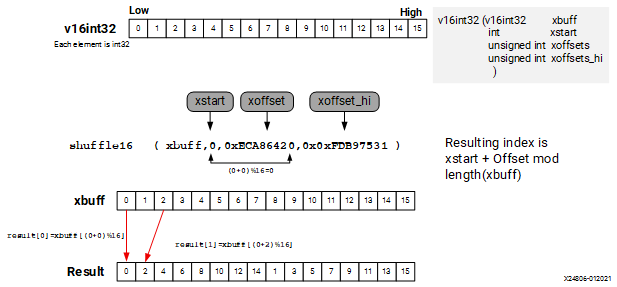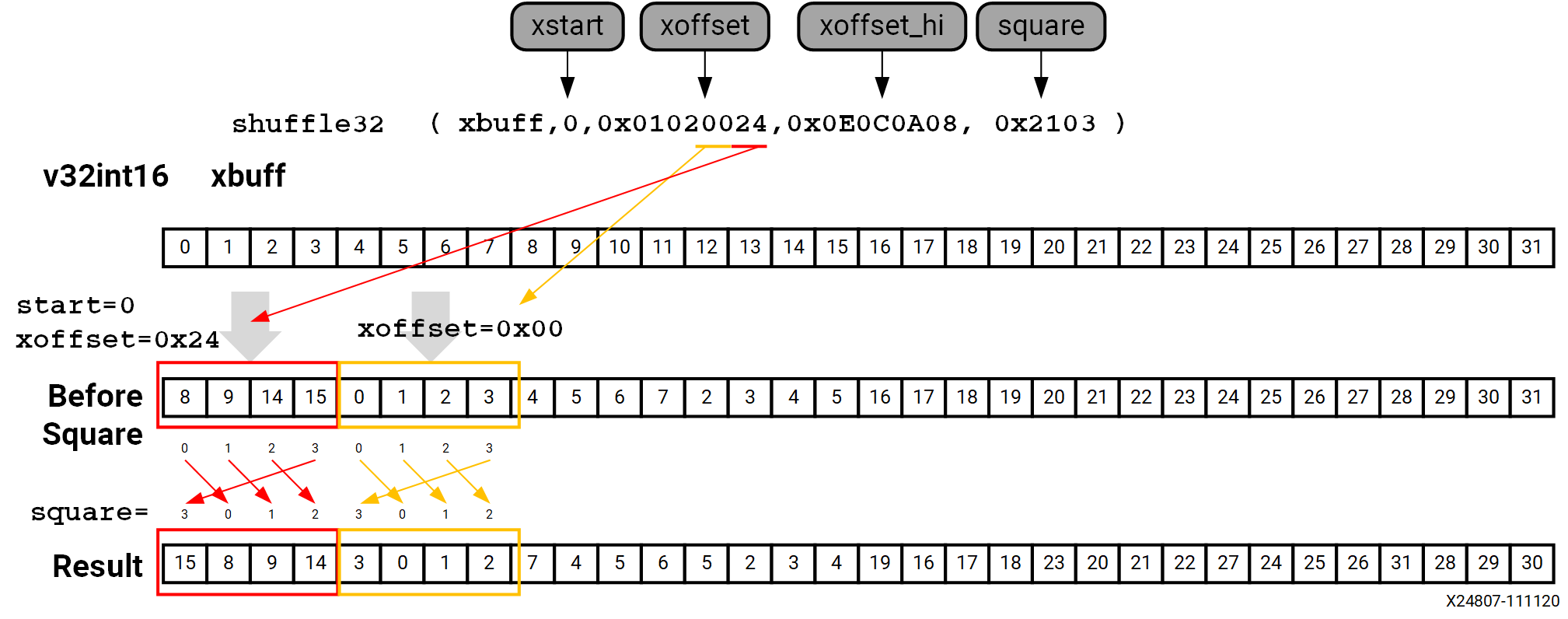# Data Shuffle - 2022.2 English

## AI Engine Kernel and Graph Programming Guide (UG1079)

Document ID
UG1079
Release Date
2022-10-19
Version
2022.2 English

The AI Engine shuffle intrinsic function selects data from a single input data buffer according to the start and offset parameters. This allows for flexible permutations of the input vector values without needing to rearrange the values. `xbuff` is the input data buffer, with `xstart` indicating the starting position offset for each lane in the `xbuff` data buffer and `xoffset` indicating the position offset applied to the data buffer. The shuffle intrinsic function is available in 8, 16, and 32 lane variants (`shuffle8`, `shuffle16`, and `shuffle32`). The main permute for data (`xoffsets`) is at 32-bit granularity and `xsquare` allows a further 16-bit granularity mini permute after main permute. Thus, the 8-bit and 16-bit vector intrinsic functions can have additional square parameter- for more complex permutations.

For example, a `shuffle16` intrinsic has the following function prototype.

``````v16int32 shuffle16	(	v16int32 	xbuff,
int 	xstart,
unsigned int 	xoffsets,
unsigned int 	xoffsets_hi
)	``````

The data permute performs in 32 bits granularity. When the data size is 32 bits or 64 bits, the start and offsets are relative to the full data width, 32 bits or 64 bits. The lane selection follows the regular lane selection scheme.

``f: result [lane number] = (xstart + xbuff [lane number]) Mod input_samples``

The following example shows how shuffle works on the `v16int32` vector. `xoffset` and `xoffset_hi` have 4 bits for each lane. This example moves the even and odd elements of the buffer into lower and higher parts of the buffer.

Figure 1. Data Shuffle on int32 TypeWhen data permute is on 16 bits data, the intrinsic function includes another parameter, `xsquare`, allowing flexibility to perform data selection in each 4 x 16 bits block of data. The `xoffset` comes in pairs. The first hex value is an absolute 32 bits offset and picks up 2 x 16 bits values (index, index+1). The second hex value is offset from first value + 1 (32 bits offset) and picks up 2 x 16 bits values. For example, `0x00` selects index 0, 1, and index 2, 3. `0x24` selects index 8, 9, and index 14, 15. Following is a shuffle example on the `v32int16` vector.

Figure 2. Data Shuffle on int16 Type